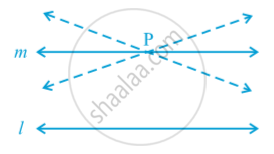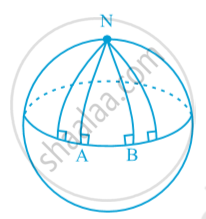# Equivalent Versions of Euclid’S Fifth Postulate

#### notes

Euclid’s fifth postulate is very significant in the history of mathematics.  There are no intersection of lines will take place when the sum of the measures of the interior angles on the same side of the falling line is exactly 180°.  There are several equivalent versions of this postulate. One of them is ‘Playfair’s Axiom’  as stated below:
‘For every line l and for every point P not lying on l, there exists a unique line m passing through P and parallel to l’. in the following fig . you can see that of all the lines passing through the point P , only line m is parallel to line l .This result can also be stated in the following form:
Two distinct intersecting lines cannot be parallel to the same line.
All the attempts to prove Euclid’s fifth postulate using the first 4 postulates failed. But they led to the discovery of several other geometries, called non-Euclidean geometries.

Many mathematician including Euclid himself thought that fifth postulate is actually a theorem.
However, all attempts to prove the fifth postulate as a theorem have failed. but these efforts have led to the creation of several other geometries . They are called  non-Euclidean geometries (Example : Spherical Geometry).In spherical geometry, lines are not straight. They are parts of great circles (i.e., circles obtained by the intersection of a sphere and planes passing through the centre of the sphere).
In fig. the lines AN and BN (which are parts of great circles of a sphere) are perpendicular to the same line AB. But they are meeting each other, though the sum of the angles on the same side of line AB is not less than two right angles (in fact, it is 90° + 90° = 180°).
Also, note that the sum of the angles of the triangle NAB is greater than 180°, as ∠ A + ∠ B = 180°.
Thus, Euclidean geometry is valid only for the figures in the plane. On the curved surfaces, it fails.

If you would like to contribute notes or other learning material, please submit them using the button below.

### Shaalaa.com

Equivalent Versions of Euclid’s Fifth Postulate [00:08:05]
S
0%